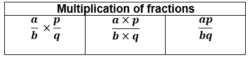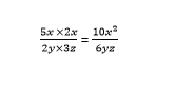# Multiplying Fractions

Multiplying fractions is as simple as multiplying any numbers in the number systems. A fraction represents the division of the whole. Fractions, when multiplied by whole numbers, can give a fraction or a whole number. But when multiplied by variables, it becomes a variable quantity. It consists of a numerator and denominator and types of fractions are proper fractions, improper fractions and mixed fractions.Multiplying Fractions

To multiply fractions, simplify it first and then multiply the numerators and denominators separately.

Multiplication of fractions also results in fractions, which has numerator and denominator as seen while dividing the fractions. There are various concepts which we can learn when it comes in terms of fractions and operations performed in it. Multiplication and Division of fractions are required to be understood properly, but addition and subtraction of them are very easy to solve. Multiplication of fractions with same denominators or different one requires the same effort unlike dividing fractions for the same given conditions.

The concept of fractions is also used in our daily life. Like if we divide an apple into 4 equal parts, then the value of each part is ¼ and if we divide it into 8 equal parts, then the value of each piece is 1/8th of the whole apple. Before learning about the multiplication of fractions, let us learn parts and types of fractions first.

Also Check: Addition And Subtraction Of Fractions

## Multiplication of Fractions

Fractions multiplication is similar to the multiplication of any two real numbers. Or you can say, we apply the simple method to multiply fractions here. Simply, we can write the formula for multiplication of fraction as;

The product of Fraction = Product of numerator/Product of denominator

Try This: Multiplying Fractions Calculator

## Types of Fraction Multiplication

Let us understand the above concepts using some example questions.

### Multiplication of Fractions with Fractions

Example 1: Solve ⅔×½

Solution: ⅔×½ = 2×1/3×2 = 2/6 =

Therefore, from the above example, we can observe, by multiplying two fractions we get a fraction number. This is a proper fraction.

Example 2: Multiply ⅘×⅞

Solution: ⅘×⅞ = 4×7 / 5×8 = 28/40

We can further simplify it as;

28/40 = 7/10

If we have to multiply three fractions, then the above formula remains the same.

Example 3: Multiply ¼×⅖×⅛

Solution: Multiplying the given fraction ¼×⅖×⅛, we get

Product = 1×2×1 / 4×5×8

= 2 / 160

= 1 / 80

### Multiplying Fractions with Whole numbers

If a whole number or real number is multiplied with fraction, then it is equal to the real number times the fraction is added.

Example 4: Multiply 5×½

Solution: 5×½ means 5 times of ½

Means, if we add ½ for five times, we get the answer.

Therefore,

½ + ½ + ½ + ½ +½ = (1+1+1+1+1)/2 = 5/2 = 2.5

Example 5: Multiply 8/7×10

Solution: Given, 8/7×10

We can write it as 8×10/7

Therefore, 80/7 is the answer. In decimal, it is 11.42.

• Note: If we multiply a mixed fraction to a whole number or real number, then we get a fraction itself.

Example 6: Multiply $3\frac{1}{5}$×12

Solution: Simplifying the value $3\frac{1}{5}$ we get,

16/5×12 = 16×12 / 5 = 192 / 5 = 38.4

### Multiplying Fractions with Variables

Now, consider the fraction is multiplied with a variable, then the results or outcome will be as per below examples.

Example 7: Multiply 5x/2y × 2x/3z

Solution: Given, 5x/2y × 2x/3z

Therefore, we can solve the above-given expression as;### How to Multiply Fractions?

To multiply fractions, first simply the fraction to its lowest term. In the case of mixed fractions, simplify it. After simplifying the fraction, multiply the numerator with the numerator and denominator with the denominator. Then, the product of fractions is obtained in p/q form.

### How to Multiply a Fraction Times a Whole Number?

To multiply a fraction with a whole number, represent the whole number as a fraction by putting 1 in the denominator. Then, multiply the numerator with the numerator and the denominator with the denominator to get the product.

### Do you Need Common Denominators to Multiply Fractions?

No, there is no need for a common denominator to multiply fractions. Any two fractions can be multiplied in which numerators are multiplied with each other and the denominators are multiplied with each other.

### How to Multiply Fractions with Mixed Numbers?

BIf a fraction has to be multiplied with a mixed number (fraction), simplify the fraction first. Once the mixed fraction is in the form of p/q, multiply the numerators with numerators and denominators with denominators.

Below is a recap of fractions and its types.

### Fractions Parts and Types

If a fraction is written in the form of a/b, then a and b are the parts of the fraction, where a is called as numerator and b is called as the denominator.

For example,

Suppose ⅖ is a fraction, then 2 is the numerator and 5 is the denominator.

There are three main types of fractions which are proper fractions, improper fractions, and mixed fractions. Below is a brief explanation on each of the types.

• Proper fractions

When numerator of a fraction is less than the denominator.

Example: ½, ⅗, 7/9

• Improper fractions

When the numerator is greater than the denominator

Example: 3/2, 5/4, 8/3

• Mixed Fraction

We can also write the improper fraction in the combination of a whole number and a fraction. This type of fraction is called a mixed fraction.

Example: 13/4 = $3\frac{1}{4}$

21/5 = $4\frac{1}{5}$

Download BYJU’S – The Learning App and have fun learning with us with interactive videos.